Next: Dielectric constant of a Up: Electromagnetic radiation Previous: Faraday rotation

## Propagation in a conductor

Consider the propagation of an electromagnetic wave through a conducting medium which obeys Ohm's law: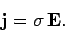(1184)

Here,is the conductivity of the medium in question. Maxwell's equations for the wave take the form: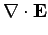(1185)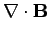(1186)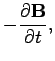(1187)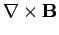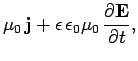(1188)

where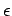is the dielectric constant of the medium. It follows, from the above equations, that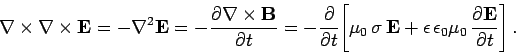(1189)

Looking for a wave-like solution of the form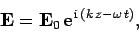(1190)

we obtain the dispersion relation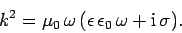(1191)

Consider a poor'' conductor for which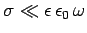. In this limit, the dispersion relation (1191) yields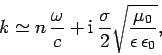(1192)

where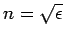is the refractive index. Substitution into Eq. (1190) gives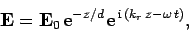(1193)

where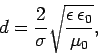(1194)

and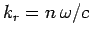. Thus, we conclude that the amplitude of an electromagnetic wave propagating through a conductor decays exponentially on some length-scale,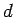, which is termed the skin-depth. Note, from Eq. (1194), that the skin-depth for a poor conductor is independent of the frequency of the wave. Note, also, that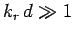for a poor conductor, indicating that the wave penetrates many wave-lengths into the conductor before decaying away.

Consider a good'' conductor for which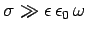. In this limit, the dispersion relation (1191) yields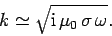(1195)

Substitution into Eq. (1190) again gives Eq. (1193), with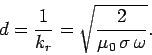(1196)

It can be seen that the skin-depth for a good conductor decreases with increasing wave frequency. The fact that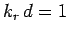indicates that the wave only penetrates a few wave-lengths into the conductor before decaying away.

Now the power per unit volume dissipated via ohmic heating in a conducting medium takes the form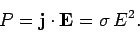(1197)

Consider an electromagnetic wave of the form (1193). The mean power dissipated per unit area in the region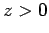is written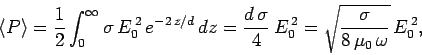(1198)

for a good conductor. Now, according to Eq. (1165), the mean electromagnetic power flux into the regiontakes the form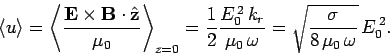(1199)

It is clear, from a comparison of the previous two equations, that all of the wave energy which flows into the regionis dissipated via ohmic heating. We thus conclude that the attenuation of an electromagnetic wave propagating through a conductor is a direct consequence of ohmic power losses.

Consider a typical metallic conductor such as copper, whose electrical conductivity at room temperature is about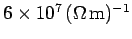. Copper, therefore, acts as a good conductor for all electromagnetic waves of frequency below about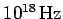. The skin-depth in copper for such waves is thus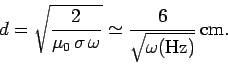(1200)

It follows that the skin-depth is about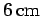at 1Hz, but only about 2mm at 1kHz. This gives rise to the so-called skin-effect in copper wires, by which an oscillating electromagnetic signal of increasing frequency, transmitted along such a wire, is confined to an increasingly narrow layer (whose thickness is of order the skin-depth) on the surface of the wire.

The conductivity of sea water is only about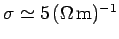. However, this is still sufficiently high for sea water to act as a good conductor for all radio frequency electromagnetic waves (i.e.,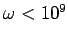Hz). The skin-depth at 1MHz (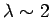km) is about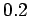m, whereas that at 1kHz (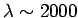km) is still only about 7m. This obviously poses quite severe restrictions for radio communication with submerged submarines. Either the submarines have to come quite close to the surface to communicate (which is dangerous), or the communication must be performed with extremely low frequency (ELF) waves (i.e.,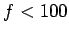Hz). Unfortunately, such waves have very large wave-lengths (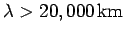), which means that they can only be efficiently generated by extremely large antennas.Next: Dielectric constant of a Up: Electromagnetic radiation Previous: Faraday rotation
Richard Fitzpatrick 2006-02-02